Next: 2.3.3 Nonlinear and Nonlocal Up: 2.3 The Magnetic Field Previous: 2.3.1 Nonlinear Effects in

## 2.3.2 Nonlinear Effects in a dx2-y2-Wave Superconductor

For a dx2-y2-wave superconductor, the presence of nodes on the Fermi surface means that the supercurrent response to a weak applied magnetic field will be nonlinear even at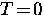. This is clearly seen in Fig. 2.4, where, due to the nodes, quasiparticle excitations will result from even a small displacement of the Fermi cylinder. For a given shift, the precise number of quasiparticle excitations will depend on the slope of the energy gap function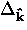at the nodes and the direction of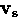. The excited quasiparticles located in a narrow wedge at the nodes produce a current density which flows in a direction opposite to that of the superfluid.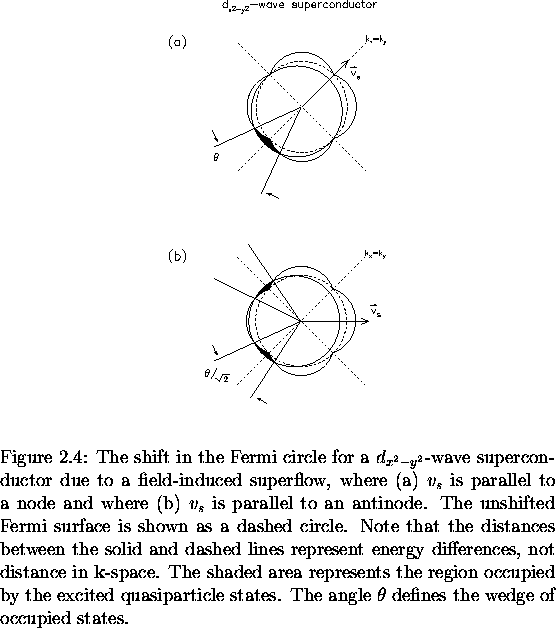For the case in whichis directed along a node, as shown in Fig. 2.4(a), the supercurrent-velocity relation is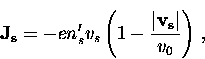(47)
where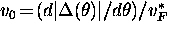, such that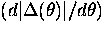, is the angular slope of the energy gap at the node, and vF* is the Fermi velocity at the node. For the case in whichis directed along an antinode, as shown in Fig. 2.4(b), the supercurrent density is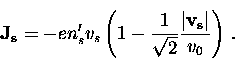(48)
The additional factor of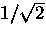is easy to understand by comparing the angular size of the wedges at the nodes in Fig. 2.4(b) to the angular size of the wedge in Fig. 2.4(a). Due to the anisotropy of the nonlinear response, Yip and Sauls  proposed that the field dependence of the penetration depth in the Meissner state could be used to resolve the structure of the energy gap in a superconductor. The magnetic penetration depth can be derived using the expressions for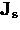. The result is that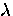changes linearly with H at low T [30,35,36]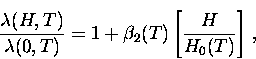(49)
where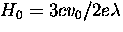and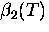is a temperature dependent coefficient which remains finite atdue to the nodes in the gap. The actual value ofwill of course depend on the direction of. The definition ofin Eq. (2.50) is the same as that in Eq. (2.47) (i.e. it is related to the initial decay rate of the field).

As the temperature is increased, there is eventually a crossover to a situation in which thermal excitation of quasiparticles also occurs away from the nodes. Below this crossover temperature T*(H),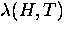is linear in H but quadratic in T, whereas above T*(H),is quadratic in H and linear in T . The first evidence for a linear H-dependence accompanied with a T2-dependence in a high-Tc material, was obtained by Maeda et al.  for measurements of the in-plane magnetic penetration depth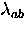in Bi2Sr2CaCu2Oy. Similar results have since been reported in YBa2Cu3O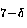and Tl2Ba2CaCu2Oy . However, the results of these experiments are suspect because of the large demagnetization effect (associated with the shape of the sample) which arises from applying a magnetic field perpendicular to the flat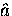-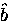plane. Early measurements ofin a single crystal of YBa2Cu3O6.95 found a large H2 term , but the sample had a reduced Tc indicating there may have been extrinsic effects due to impurities.Next: 2.3.3 Nonlinear and Nonlocal Up: 2.3 The Magnetic Field Previous: 2.3.1 Nonlinear Effects in